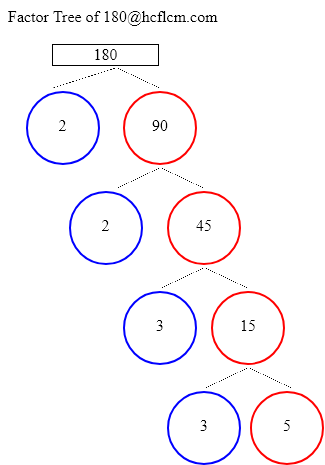# Factors of 180 | Find the Factors of 180 by Factoring Calculator

Factoring Calculator calculates the factors and factor pairs of positive integers.

Factors of 180 can be calculated quickly with the help of Factoring Calculator i.e. 1, 2, 3, 4, 5, 6, 9, 10, 12, 15, 18, 20, 30, 36, 45, 60, 90, 180 positive integers that divide 180 without a remainder.

Factors of 180 are 1, 2, 3, 4, 5, 6, 9, 10, 12, 15, 18, 20, 30, 36, 45, 60, 90, 180. There are 18 integers that are factors of 180. The biggest factor of 180 is 180.

Factors of:

### Factor Tree of 180 to Calculate the Factors

 180 2 90 2 45 3 15 3 5Factors of 180 are 1, 2, 3, 4, 5, 6, 9, 10, 12, 15, 18, 20, 30, 36, 45, 60, 90, 180. There are 18 integers that are factors of 180. The biggest factor of 180 is 180.

Positive integers that divides 180 without a remainder are listed below.

• 1
• 2
• 3
• 4
• 5
• 6
• 9
• 10
• 12
• 15
• 18
• 20
• 30
• 36
• 45
• 60
• 90
• 180

### Factors of 180 in pairs

• 1 × 180 = 180
• 2 × 90 = 180
• 3 × 60 = 180
• 4 × 45 = 180
• 5 × 36 = 180
• 6 × 30 = 180
• 9 × 20 = 180
• 10 × 18 = 180
• 12 × 15 = 180
• 15 × 12 = 180
• 18 × 10 = 180
• 20 × 9 = 180
• 30 × 6 = 180
• 36 × 5 = 180
• 45 × 4 = 180
• 60 × 3 = 180
• 90 × 2 = 180
• 180 × 1 = 180

### Factors of 180 Table

FactorFactor Number
1one
2two
3three
4four
5five
6six
9nine
10ten
12twelve
15fifteen
18eighteen
20twenty
30thirty
36thirty six
45forty five
60sixty
90ninety
180one hundred eighty

### How to find Factors of 180?

As we know factors of 180 are all the numbers that can exactly divide the number 180 simply divide 180 by all the numbers up to 180 to see the ones that result in zero remainders. Numbers that divide without remainder are factors and in this case below are the factors

1, 2, 3, 4, 5, 6, 9, 10, 12, 15, 18, 20, 30, 36, 45, 60, 90, 180 are the factors and all of them can exactly divide number 180.

### Frequently Asked Questions on Factors of 180

1. What are the factors of 180?

Answer: Factors of 180 are the numbers that leave a remainder zero. The ones that can divide 180 exactly i.e. factors are 1, 2, 3, 4, 5, 6, 9, 10, 12, 15, 18, 20, 30, 36, 45, 60, 90, 180.

2.What are Factor Pairs of 180?

Answer:Factor Pairs of 180 are

• 1 × 180 = 180
• 2 × 90 = 180
• 3 × 60 = 180
• 4 × 45 = 180
• 5 × 36 = 180
• 6 × 30 = 180
• 9 × 20 = 180
• 10 × 18 = 180
• 12 × 15 = 180
• 15 × 12 = 180
• 18 × 10 = 180
• 20 × 9 = 180
• 30 × 6 = 180
• 36 × 5 = 180
• 45 × 4 = 180
• 60 × 3 = 180
• 90 × 2 = 180
• 180 × 1 = 180

3. What is meant by Factor Pairs?

Answer:Factor Pairs are numbers that when multiplied together will result in a given product.